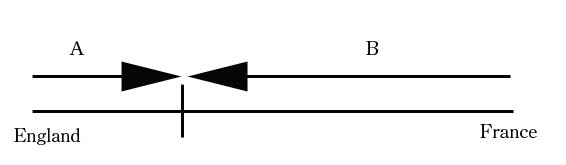SEARCH HOMEMath Central Quandaries & QueriesQuestion from Elisha, a student: Trains at each end of the 50. km long Eurotunnel under the English Channel start at the same time into the tunnel. Find their speeds if the train from France travels 8. km/h faster than the train from England and they pass in 17 minutes.We have two responses for you

Elisha,

The important fact here is that rate = distance/time or in other words distance = time × rate.

Suppose the train from England travels at e km/h then the train from France travels at e + 8 km/h. Suppose also that the train from England travels d km in 17 minutes. Since the trains meet at this time the train from France must travel 50 - d km in 17 minutes. In this description I used time in hours at one point and time in minutes at another. I need to use the same units in both places and I decided to use hours so I will use 17 minutes = 17/60 hours.

From distance = time × rate for the train from England I get

d = 17/60 × e

Write a similar expression for the train from France. This gives you two equations in d and e. Solve for e.

Penny

Hi Elisha,

Since we do not know where the trains pass each other, we will choose an arbitrary point:The distance from England to the passing point is A and the distance from France to the passing point is B. If we let the velocity of the train traveling from England be v then the train traveling from France will be v+8. Remember that since our speed is in km/h, we need to have consistent units so time=17/60 hour. We know A +B=50km and distance = velocity x time so

(17/60)v +(17/60)(v+8)=50

Now solve for v and you will have the speed of the train traveling from England.Math Central is supported by the University of Regina and The Pacific Institute for the Mathematical Sciences.